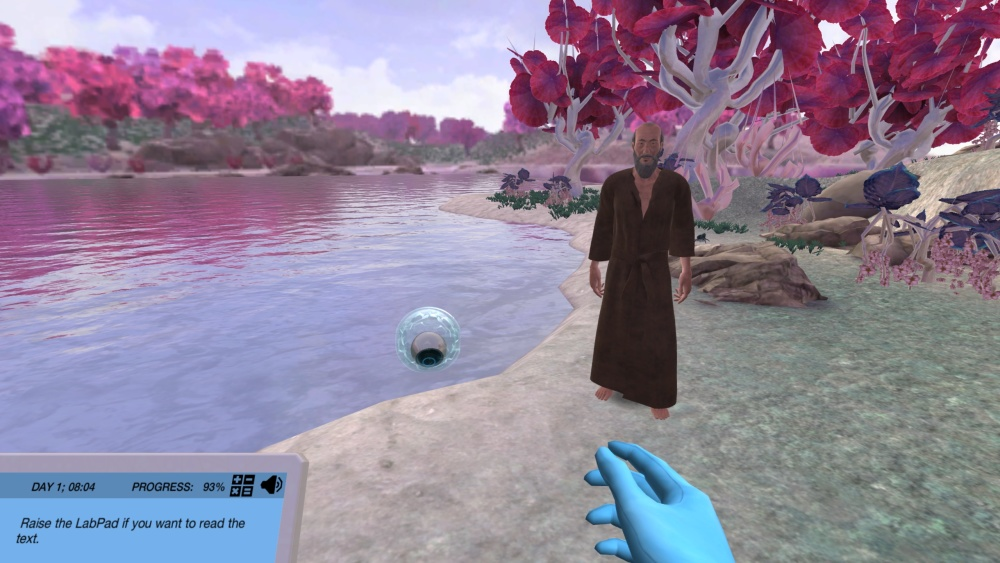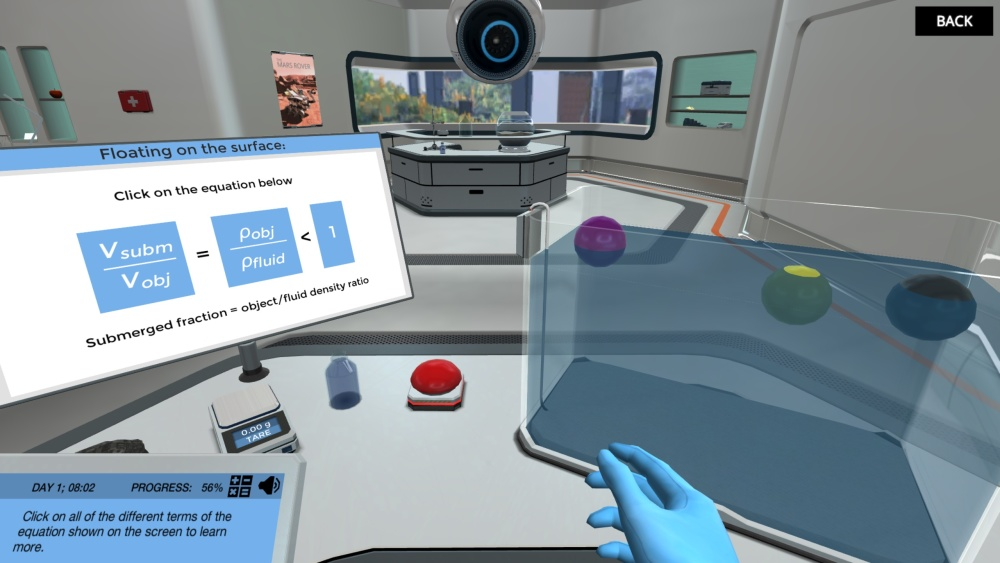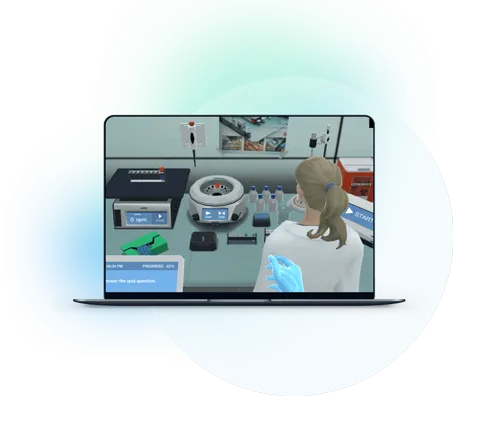Blog

# 5 Ways to Make Buoyancy a More Approachable Topic for Students

Teaching with Labster
October 11, 2022

All our activities in the water are governed by the force of buoyancy. It is the buoyant force that makes it possible to travel in boats, ships, and submarines or just enjoy a relaxing dip in a lake. In short, we need the liquid's upward force, buoyancy, to keep us afloat.

The concept of Buoyancy was first given by Greek scientist Archimedes who said that if the object is fully or partially submerged in a liquid, it experiences an upward force whose magnitude is equivalent to the weight of the liquid displaced. This makes sense because, prior to the object being inserted into the fluid, the same volume of fluid was in balance with its surroundings.

With so many practical applications in daily life, this concept is considered an important one in Fluid Mechanics.

For some students, the concept can be quite trickier to comprehend. However, using some techniques, you can add more crispness to the subject and make it approachable for students.

Read on to learn why it can be trickier for students and teachers, and five techniques that will make the topic interesting.GIF from Labster's Applications of Buoyancy simulation, available at the high school level.

## Why is the Concept of Buoyancy Trickier for Students?

Physics is quite interesting compared to other sciences since it closely explains the functioning principles of physical phenomena around us. However, some topics like Buoyancy and Archimedes principle can be trickier for students.

### 1. Multiple Concepts Overlap

In early science classes, students are told that objects which are less dense than the fluid, float on them. This is true. However, when you teach them the concept of Buoyancy and Archimedes principle. At times, they fail to find the relation between the two concepts despite the fact that both these concepts are synonymous.

### 2. Force Diagrams Can be Tricky

In Physics, whenever we explain the motion of an object, whether it is a car moving on the road, or a ship floating on an ocean, we draw force diagrams. These force diagrams help us to simplify the problem and understand in which direction will the motion take place.

For students, marking down all the forces and drawing them on the sheet can be trickier. At times, they do not understand all the acting forces involved. For instance, in this case, a force diagram will contain the buoyancy force and the weight of the object.

### 3. It’s Content Heavy

With the concept of Buoyancy, you need to explain the Archimedes principle or flotation principle and then correlate it with the students’ previous concepts. The force diagrams, and determining how objects float and sink in water, make it a lot for students to digest in one go.

## 5 Ways to Make Buoyancy easy-to-understand Topic

Keeping in view above mentioned things, here are five ways through which you can make buoyancy and its principle more fun and exciting for students.

### 1. Tell them the interesting story of Archimedes

How easy the topic may be, starting it with an interesting story of the scientist who discovered it is always a good idea.  Moreover, you can tell the tale during your lecture whenever it gets boring for students. The storytelling makes them pay attention to the topic and helps in remembering it in the long term.

The tale of how Archimedes got the idea of buoyancy is very intriguing just like Newton’s story of a falling apple. Archimedes was a Greek mathematician and physicist born in 287 BC. One day, the king, Heiro II, hired him to determine if his crown was made out of pure gold or silver.

While he was pondering to solve this problem in the bathroom, the water just pushed out of the bathtub as he stepped into it. From this incident, he concluded that when an object is submerged in fluid water it displaces a volume of fluid equal to the object’s volume. He got so excited from an incident that he ran naked into the streets shouting “Eureka” (I have found it.)

Archimedes then used the same concept to check the authenticity of the crown. He took equal masses of gold and silver and put them in a vessel filled with water one by one. He noted down the volume of water displaced by each mass.  The silver mass displaced out more water than the gold one. When he put the crown in the water vessel, it displaced more water than gold mass but less than that of silver.  As a result, he concluded the crown wasn’t made out of pure gold.

### 2. Explain the Basic Things First and then Move on to Complex ones

Start off things simply. Even if the students have prior ideas about the concept, you just need to start your lecture from the basics. This clears out all the prior misconceptions that students might have and they get a fresh start.

Start your explanation with the buoyant force. Tell students that when an object is fully or partially submerged in a fluid, it experiences an upward force, known as the force of buoyancy. This force is equal to the weight of the fluid displaced by the object. Since the weight of the object is acting downward, the reaction force known as the buoyant force will act upward. To illustrate you can draw a simple force diagram.

Students have misconceptions that the buoyant force depends on the material and shape of the object. However, it just depends on two things, the density of the fluid and the volume of the submerged portion. That is why a solid metal ball and a hollow plastic of the same volumes will experience the same force of buoyancy.

Once you have explained the buoyancy and Archimedes, it is easy for you to tell them why objects sink and float. If the weight of the object is less than the force of buoyancy the object floats, and when it is greater, the object sinks.

### 3. Mathematically Prove the Theoretical Concepts

In earlier classes, we were told that things with densities less than water float on water. That is absolutely true. In fact, you can deduce this result from the Archimedes principle if you simplify the Archimedes principle’s expression, a little more.

According to the principle of flotation, the object floats on the fluid if its weight is less than the force of buoyancy. Mathematically, it is written as:

m · g < FB

Since the force of buoyancy, FB = ρfluid · Vobject · g. So, the equation becomes:

mobject · g < ρfluid · Vobject · g

If we cancel out the “g” term on both sides, then we get:

mobject < ρfluid · Vobject

Moving volume to the left side, we get:

mobject / Vobject < ρfluid

mobject / Vobjectis the density of object ρobject. So, our final equation becomes:

ρobject < ρfluid

So, our prior concept that objects float due to differences in densities is actually derived from Archimedes' principle.

### 4. Relate it to the real world- Mention Applications

Teaching Physics without telling students how the law is used in our daily applications is useless. If they don’t understand how the theoretical concept is useful in application, they take no interest in studying it.

In this case, you have to lot of applications to mention. The whole flotation principle is based upon the Archimedes principle. The boats, ships, and submarines, all are designed based on the calculations of Archimedes' principle.

The most asked question by high-school students is, “Why a small block of iron would sink in water but huge cargo ships, weighing thousands of tons float on water?”

The answer is simple; all ships are designed to maximize the buoyancy force acting on them. To achieve that, the ship’s bottom (hull) is made hollow. It usually occupies most of the ship's volume. As the volume increases, the ship’s overall density (mass per unit volume) becomes lesser than water. So, it floats on water.

You can also put it in this way; the more the ship’s volume, the greater amount of water is displaced. Hence, a larger force of buoyancy.

We can also determine the densities of unknown objects using the Archimedes principle, just as Archimedes determined if the crown was made of gold.A snippet from Labster's Applications of Buoyancy simulation.

### 5. Use Simulations

Lastly, the easiest way to explain complex and practical things is the use of computer simulation. You don’t need any costly equipment to experiment. You can just use the virtual lab to explain things to your pupils.

Here is where our program, Labster, can be of great use. At Labster, we're committed to creating interactive lab simulations that make use of gamification components with stories and score systems, all set in an engrossing, 3D world.Check out Labster’s Applications of Buoyancy simulation where students learn about the buoyancy concept through an experiment. In the simulation, students place different weighted balls in a tank filled with lake water. They investigate how densities and fluid affect the force of buoyancy, and what causes objects to float or sink.What exactly are virtual labs?